This site requires JavaScript to be enabled
IE BUMPERTosca Version
Incident
Language
How can we help?

### Solution SuggestionsIE BUMPER
Categories
Boolean Operators
OR or vertical bar symbol (|)
Finds a match if either the terms exist in a document (a union using sets).
AND
Finds a match if both terms exist in a document (an intersection of sets).
NOT, minus (-), or exclamation point (!)
Excludes documents that contain the term after NOT (a difference of sets).
Wildcards
Asterisk (*)
The asterisk symbol performs a multi character wildcard search.
Percent sign (%)
The percent sign performs a single character wildcard search.
Phrases
Quotation marks (")
Use quotation marks to search for an exact phrase with multiple terms.

#### Copy Excel data from one worksheet/workbook to another worksheet/workbook

Copy Excel data

This article contains key examples when user wants to buffer excel data in multiple different buffers for further evaluation on the values. Also, this article would help user when trying to copy data from one worksheet/workbook to another worksheet/workbook.

# Situation

1) How to Copy whole Excel table in different buffers (irrespective of No. of Rows and Columns).

2) How to copy whole Excel table from one worksheet to another worksheet of same Workbook.

3) How to copy whole Excel table in different workbook.

# Solution

All use cases can be achieved using loops shown as below. Also, please import the Tosca AidPack as per the Tosca version being used.

## 1. Copy whole Excel table values in different buffers excluding the Header content of the table

Input Table: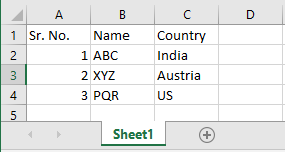1. Open the Excel workbook by using "Excel open workbook" module from Aidpack and provide the path along with file name where excel file is stored.

2. Use "Excel Range Manipulation" module to get RowCount and ColumnCount as shown below. Number of columns will be stored in columnsnum buffer and Number of rows will be stored in rowsnum buffer.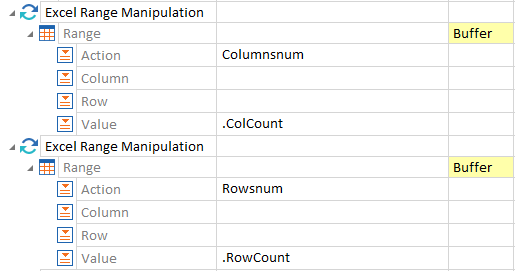3. Use "Excel Create Range" module and provide value for Startcell.Row as per the starting row of the table. In this example, this will ensure that table starts from 1st Row and 1st column.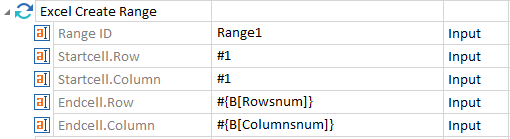4. Initialize counter buffer i with value as 1.  Use Excel Range Manipulation module under nested Repetition folders to create unique buffers. As per our use case, it will create buffers as BufA_1_1, BufA_1_2 and so on.

Below Repetition property should be applied to the outer and inner Repetition folder:

Outer Folder : Repetition = {MATH[{B[Rowsnum]}-1]}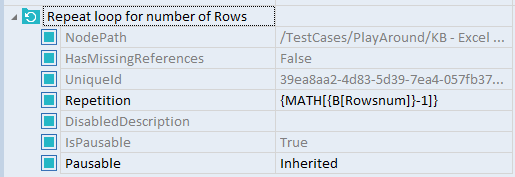Inner folder : Repetition = {B[Columnsnum]}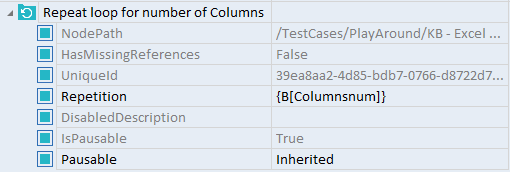Logic as follows: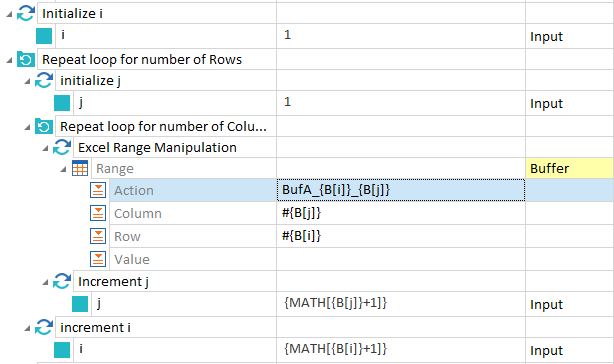5. Use close workbook module to close the workbook. Set "Save Workbook" attribute value as true.

## 2. Copy Excel table from one worksheet to another worksheet of same Workbook

In order to achieve this use case, we need to first save the table values into different buffers (Which we did in section 1) and then use those buffers as input to another worksheet of the same Workbook. Below is the step by step information which need to be used  while performing this use case.

1. Open Excel workbook

2. Use "Set Active Worksheet" module to set the current worksheet as Active.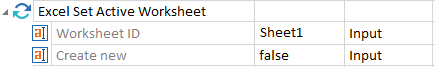3. Use "Excel Range manipulation" module to get the Row count and Column count of the table as per Section 1.

4. Use Excel Create Range module to set the active range of the table as per Section 1.

5. Use the same logic module which was used in section 1 and buffer all the excel data.

6. Close the worbook and make sure Save Workbook is set to true.

7. Open the same Excel workbook by using Open Excel workbook module

8. Use "Set Active Worksheet" module to set the current worksheet as Active. Please note that if new sheet is not created manually, set "Create new" attribute as True and if new sheet already exists, then it should be set as false.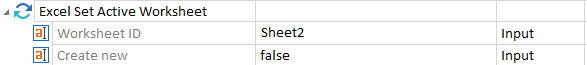9. Use the same logic module which was used in Step 5 and set Actionmode to Input in Excel Range Manipulation module mentioned as below. Do not forget to add Repetition properties for both the folders.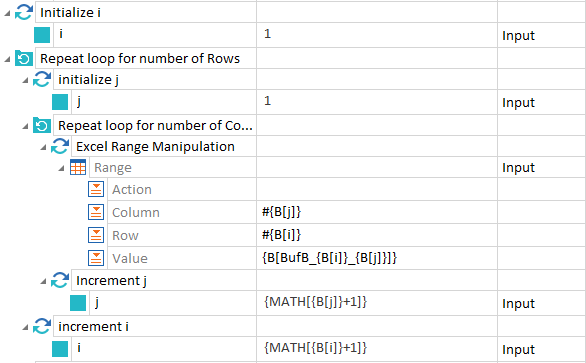10. Close the excel workbook. Set "Save Workbook" attribute value as true.

## 3. Copy whole Excel table in different workbook

This section provides step-by-step information on how to copy a table from one workbook to another workbook. We need to use 1 additional module while writing the values to the second Excel workbook.

1. Open Excel workbook 1 (In our example we have used test_excel.xlsx)

2. Use "Set Active Worksheet" module to set the current worksheet as Active.

3. Use Excel Range manipulation module to get the Row count and Column count of the table

4. Use Excel Create Range module to set the active range of the table

5. Use the same logic module which was used in section 1

6. Close the worbook and make sure Save Workbook is set to true

7. Open the other Excel workbook (As per our example, file name is copy_excel.xlsx). Please make sure that this excel sheet is already created as "Create New" attribute is set to False here.

8. Use module Excel Set Active Workbook to set the second workbook as Active

9. Use "Set Active Worksheet" module to set the current worksheet as Active.

10. Use the same logic module which was used in Step 9 of Section  and set Actionmode to Input in Excel Range Manipulation module

11. Close the excel workbook. Set "Save Workbook" attribute value as true.